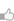求知 文章 文库 Lib 视频 iProcess 课程 认证 咨询 工具 讲座 Modeler Code

 Python教程 Python快速入门 Python概述 Python环境安装 Python基本语法 Python变量类型 Python基本运算符 Python决策 Python循环 Python数据类型 Python字符串 Python列表 Python元组 Python字典 Python日期时间Date/Time Python函数 Python模块 Python文件I/O Python异常处理 高级教程 Python 3开发网络爬虫(一) Python 3开发网络爬虫(二) Python 3(三): 伪装浏览器 Python 3(四): 登录 Python面向对象 Python正则表达式 Python CGI编程
Python函数
 463 次浏览23次捐助

 def functionname( parameters ): "function_docstring" function_suite return [expression]

 def printme( str ): "This prints a passed string into this function" print str return

 #!/usr/bin/python # Function definition is here def printme( str ): "This prints a passed string into this function" print str; return; # Now you can call printme function printme("I'm first call to user defined function!"); printme("Again second call to the same function");

 I'm first call to user defined function! Again second call to the same function

 #!/usr/bin/python # Function definition is here def changeme( mylist ): "This changes a passed list into this function" mylist.append([1,2,3,4]); print "Values inside the function: ", mylist return # Now you can call changeme function mylist = [10,20,30]; changeme( mylist ); print "Values outside the function: ", mylist

 Values inside the function: [10, 20, 30, [1, 2, 3, 4]] Values outside the function: [10, 20, 30, [1, 2, 3, 4]]

 #!/usr/bin/python # Function definition is here def changeme( mylist ): "This changes a passed list into this function" mylist = [1,2,3,4]; # This would assig new reference in mylist print "Values inside the function: ", mylist return # Now you can call changeme function mylist = [10,20,30]; changeme( mylist ); print "Values outside the function: ", mylist

 Values inside the function: [1, 2, 3, 4] Values outside the function: [10, 20, 30]

 #!/usr/bin/python # Function definition is here def printme( str ): "This prints a passed string into this function" print str; return; # Now you can call printme function printme();

 Traceback (most recent call last): File "test.py", line 11, in printme(); TypeError: printme() takes exactly 1 argument (0 given)

 #!/usr/bin/python # Function definition is here def printme( str ): "This prints a passed string into this function" print str; return; # Now you can call printme function printme( str = "My string");

 My string

 #!/usr/bin/python # Function definition is here def printinfo( name, age ): "This prints a passed info into this function" print "Name: ", name; print "Age ", age; return; # Now you can call printinfo function printinfo( age=50, name="miki" );

 Name: miki Age 50

 #!/usr/bin/python # Function definition is here def printinfo( name, age = 35 ): "This prints a passed info into this function" print "Name: ", name; print "Age ", age; return; # Now you can call printinfo function printinfo( age=50, name="miki" ); printinfo( name="miki" );

 Name: miki Age 50 Name: miki Age 35

 def functionname([formal_args,] *var_args_tuple ): "function_docstring" function_suite return [expression]

 #!/usr/bin/python # Function definition is here def printinfo( arg1, *vartuple ): "This prints a variable passed arguments" print "Output is: " print arg1 for var in vartuple: print var return; # Now you can call printinfo function printinfo( 10 ); printinfo( 70, 60, 50 );

 Output is: 10 Output is: 70 60 50

Lambda形式可以采取任何数量的参数，但在表现形式上只返回一个值。它们不能包含命令或多个表达式。

lambda函数都有自己的命名空间，并且不能访问变量高于在其参数列表和那些在全局命名空间等。

lambda函数的语法仅包含单个语句，如下：

 lambda [arg1 [,arg2,.....argn]]:expression

 #!/usr/bin/python # Function definition is here sum = lambda arg1, arg2: arg1 + arg2; # Now you can call sum as a function print "Value of total : ", sum( 10, 20 ) print "Value of total : ", sum( 20, 20 )

 Value of total : 30 Value of total : 40

return语句：

 #!/usr/bin/python # Function definition is here def sum( arg1, arg2 ): # Add both the parameters and return them." total = arg1 + arg2 print "Inside the function : ", total return total; # Now you can call sum function total = sum( 10, 20 ); print "Outside the function : ", total

 Inside the function : 30 Outside the function : 30

 #!/usr/bin/python total = 0; # This is global variable. # Function definition is here def sum( arg1, arg2 ): # Add both the parameters and return them." total = arg1 + arg2; # Here total is local variable. print "Inside the function local total : ", total return total; # Now you can call sum function sum( 10, 20 ); print "Outside the function global total : ", total

 Inside the function local total : 30 Outside the function global total : 0
 您可以捐助，支持我们的公益事业。 1元 10元 50元 认证码：必填
 463 次浏览23次捐助